This is “Issuing and Accounting for Serial Bonds”, section 14.5 from the book Business Accounting (v. 2.0). For details on it (including licensing), click here.

For more information on the source of this book, or why it is available for free, please see the project's home page. You can browse or download additional books there. To download a .zip file containing this book to use offline, simply click here.

Has this book helped you? Consider passing it on:
Creative Commons supports free culture from music to education. Their licenses helped make this book available to you.
DonorsChoose.org helps people like you help teachers fund their classroom projects, from art supplies to books to calculators.

## 14.5 Issuing and Accounting for Serial Bonds

### Learning Objectives

At the end of this section, students should be able to meet the following objectives:

1. Define a serial bond.
2. Identify the steps to calculate the price of a serial bond and provide the proper accounting for the issuance.
3. Record the interest and payments on a serial bond over its life.
4. Explain the determination of interest expense for a serial bond and the amount that must be compounded each period.

## Recording the Issuance of a Serial Bond

Question: The previous section examined the recording of term bonds. Interest was paid each period but no payment was made on the face value until the maturity date. How do these procedures differ for a serial bond where both interest and a portion of the face value are paid periodically?

To demonstrate this process, assume that Smith Corporation issues a four-year, \$1 million serial bond on January 1, Year One. This bond will pay a 5 percent stated interest rate at the end of each year on the unpaid face value for the period. The indenture further specifies that \$250,000 of the face value is to be paid annually at the same time as the interest. Smith officials negotiate with potential investors and finally agree on a 6 percent annual effective rate. What accounting is appropriate for a serial bond?

Answer: From the previous coverage, the reporting of a term bond where the stated cash interest and the effective rate are different can be divided into five steps:

1. The cash flows required by the bond contract are identified.
2. The total present value of these cash flows is determined using the effective rate of interest negotiated by the parties. Present value mathematically removes all future interest at the appropriate rate leaving only the principal of the debt. Thus, this resulting figure is the exact amount to be paid so that the agreed-upon interest rate is earned over the life of the bond.
3. The bond is recorded at the principal (present value) amount paid by the investors.
4. The debtor pays the stated rate of interest periodically on the dates indicated in the contract.
5. The effective rate method is applied. Interest to be reported for each period is determined by multiplying the liability’s principal balance times the effective interest rate. The cash interest figure is adjusted to this calculated amount with the difference compounded (added to the principal).The series of steps shown here is also used when a bond is issued at a premium above face value. If the effective interest rate negotiated by the parties is below the stated cash rate, the amount paid for the bond (the present value) will be above face value rather than below. In effect, the high rate of cash interest makes the bond more valuable. Thereafter, the effective interest recognized each period will be below the cash interest. Adjustment is made to lower the cash interest rate to the effective rate, which also reduces the reported principal balance moving it toward face value. Thus, when the negotiated rate is below the stated cash rate, a premium is created rather than a discount. The subsequent accounting process is not affected except that the increases and decreases are reversed from the examples shown here for a discount.

This same process is applied when a serial bond is issued. The sole difference is that regular payments are also made to reduce the face value of the debt over time. To account for the Smith Corporation serial bond described above, the following five steps are required.

Identify cash flows specified in the bond contract. As shown in Figure 14.16 "Cash Payments Required by Serial Bond Contract", Smith is required to make an annual \$250,000 payment to reduce the face value of this serial bond. In addition, interest must be paid each year. During Year One, the unpaid face value is the original \$1 million. The stated rate is 5 percent necessitating a \$50,000 year-end interest payment (\$1,000,000 × 5 percent).

Following the \$250,000 payment on December 31, Year One, the face value of the bond drops to \$750,000 throughout the second year. Consequently, the interest payment at the end of Year Two is only \$37,500 (\$750,000 × 5 percent). As a serial bond, the annual payments cause the face value to get smaller so that the interest payments are less each year.

Based on the terms of the contract, the cash flows required by this bond are identified in Figure 14.16 "Cash Payments Required by Serial Bond Contract".

Figure 14.16 Cash Payments Required by Serial Bond Contract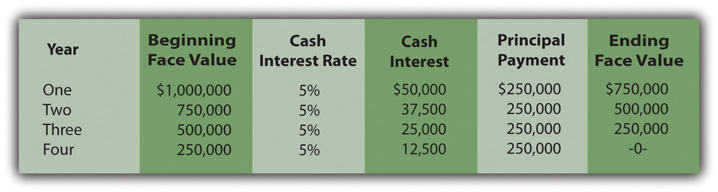Determine present value of the cash flows. These required cash flows can be organized in either of two ways.

The same cash flows are described in both cases. Thus, as proven by a comparison of Figure 14.17 "Computation of Present Value of Serial Bond—First Pattern of Cash Flows" and Figure 14.18 "Computation of Present Value of Serial Bond—Second Pattern of Cash Flows", the resulting present value will be identical (\$977,714) regardless of the approach that is followed. A link to the tables for the present value of an ordinary annuity of \$1 and the present value of \$1 are provided here but those tables are also included at the end of this textbook.

### Present Value of an Ordinary Annuity of \$1

http://www.principlesofaccounting.com/ART/fv.pv.tables/pvofordinaryannuity.htm

### Present Value of \$1

http://www.principlesofaccounting.com/ART/fv.pv.tables/pvof1.htm

Figure 14.17 Computation of Present Value of Serial Bond—First Pattern of Cash Flows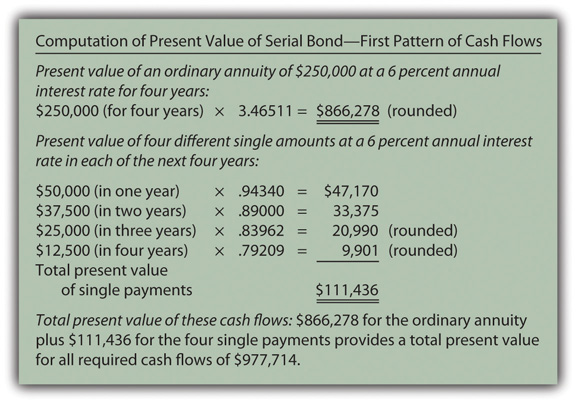Figure 14.18 Computation of Present Value of Serial Bond—Second Pattern of Cash Flows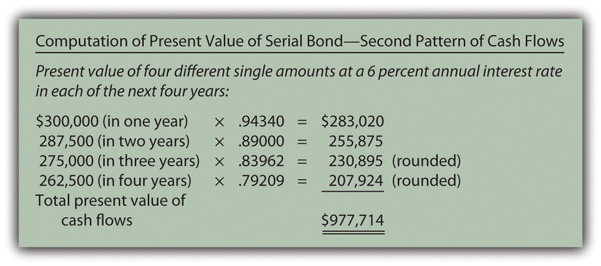Record the principal amount received in the issuance of the bond. Based on either of these computations, \$977,714 in cash is exchanged for this four-year \$1 million serial bond with an annual stated rate of 5 percent. That payment amount creates an effective rate of interest of 6 percent per year. The issuance of the bond is recorded through the journal entry shown in Figure 14.19 "January 1, Year One—Issuance of \$1 Million Serial Bonds Paying 5 Percent Annual Interest with an Effective Rate of 6 Percent".

Figure 14.19 January 1, Year One—Issuance of \$1 Million Serial Bonds Paying 5 Percent Annual Interest with an Effective Rate of 6 Percent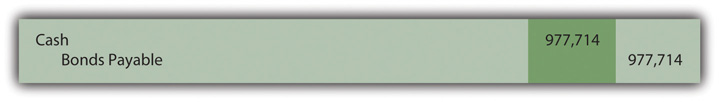Payment of stated cash interest at 5 percent annual rate. Because of the terms specified in the bond contract, interest of \$50,000 will be paid at the end of Year One, \$37,500 at the end of Year Two, and so on. The Year One interest payment is recorded in Figure 14.20 "December 31, Year One—Payment of 5 Percent Interest on Serial Bond". To better illustrate the handling of interest in a serial bond, the \$250,000 payments on the face value are shown separately in Figure 14.22 "December 31, Year One—Payment on Face Value of Serial Bond".

Figure 14.20 December 31, Year One—Payment of 5 Percent Interest on Serial Bond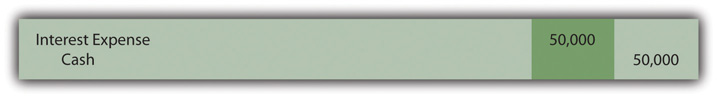Effective rate method is applied to recognize the interest rate that was negotiated by the two parties. For the first year, the principal balance of this debt is the original issuance price of \$977,714. The yield rate agreed on by the two parties was 6 percent. Thus, the interest to be recognized for Year One is \$58,663 (\$977,714 × 6 percent).

As shown in Figure 14.20 "December 31, Year One—Payment of 5 Percent Interest on Serial Bond", the cash interest paid is only 5 percent of the face value or \$50,000. The \$8,663 in extra interest for the period (\$58,663 less \$50,000) is compounded—added to the principal of the bond payable. The interest to be compounded is added to the principal in Figure 14.21 "December 31, Year One—Adjustment of Interest from Cash Rate to Effective Rate".

The journal entries shown in Figure 14.20 "December 31, Year One—Payment of 5 Percent Interest on Serial Bond" and Figure 14.21 "December 31, Year One—Adjustment of Interest from Cash Rate to Effective Rate" are often combined in practice. They are separated here for discussion purposes.

Figure 14.21 December 31, Year One—Adjustment of Interest from Cash Rate to Effective Rate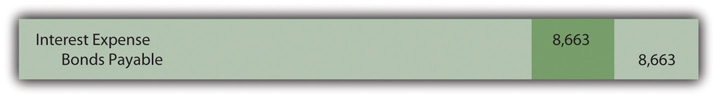In addition, as a serial bond, the first payment of the face value is made at the end of Year One and shown in Figure 14.22 "December 31, Year One—Payment on Face Value of Serial Bond".

Figure 14.22 December 31, Year One—Payment on Face Value of Serial Bond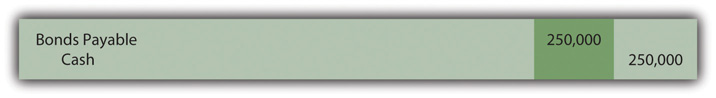Whether a long-term liability is a term bond or a serial bond, the accounting process is the same. It follows the five steps that have been listed above and demonstrated here. All the amounts to be recorded over the four-year life of this bond can be computed to verify that the final payment does appropriately remove the remainder of the debt. Those figures are presented in Figure 14.23 "Balances to be Reported Over the Four-year Life of Serial Bond".

Figure 14.23 Balances to be Reported Over the Four-year Life of Serial BondThe interest recognized in the final year has been adjusted by \$3 to compensate for the rounding of several computations so that the liability balance drops precisely to zero at the end of the four years.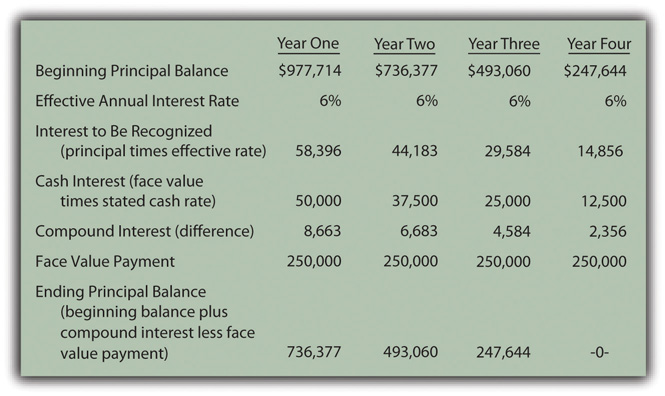### Test Yourself

Question:

The Heyman Company issues a \$500,000 serial bond on January 1, Year One. At the end of each year, the bond pays \$100,000 of the face value plus interest at a 4 percent rate on the unpaid balance for the period. The bond was sold at an effective yield rate of 5 percent per year. The present value of \$1 at a 5 percent annual rate in one year is \$0.95238, in two years is \$0.90703, in three years is \$0.86384, in four years is \$0.82270, and in five years is \$0.78353. The present value of an ordinary annuity at a 5 percent annual rate for five years is \$4.32948. What amount did Heyman Company receive when the bond was issued (rounded)?

1. \$483,865
2. \$486,590
3. \$490,130
4. \$492,545

Answer:

The correct answer is choice b: \$486,590.

Explanation:

As a serial bond, cash payments of \$100,000 are made each year. In addition, interest payments for each year are as follows.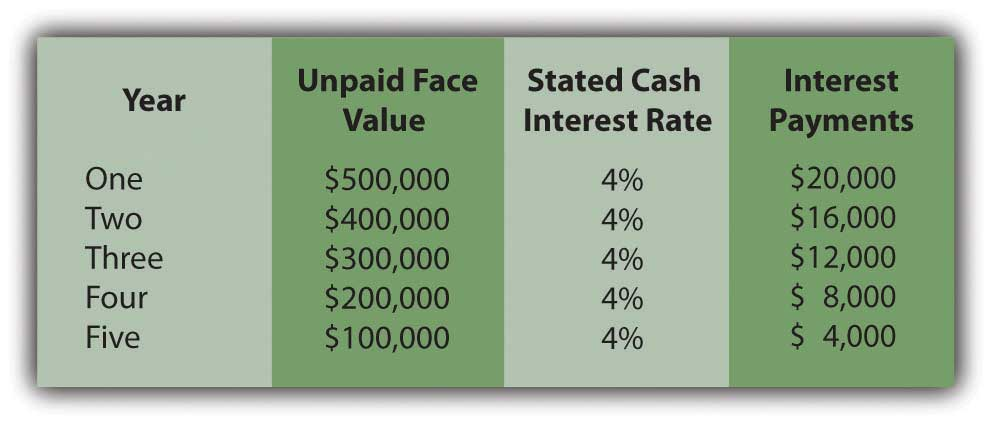By combining the face value payments with the interest, the present value at an annual rate of 5 percent can be calculated.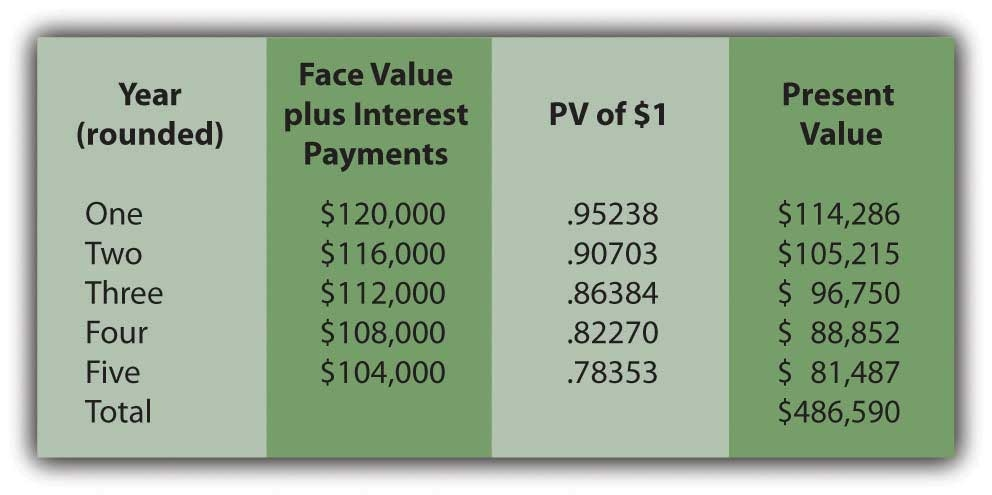### Test Yourself

Question:

The Mullins Company issues a \$500,000 serial bond on January 1, Year One. At the end of each year, the bond pays \$100,000 of the face value plus interest at a 3 percent rate on the unpaid balance for the period. The bond was sold for \$473,200 to yield an effective rate of 5 percent per year. What will Mullins report for this bond on its December 31, Year Two, balance sheet?

1. \$288,953
2. \$292,875
3. \$295,445
4. \$297,905

Answer:

The correct answer is choice a: \$288,953.

Explanation:

Effective interest for Year One is \$473,200 × the yield rate of 5 percent or \$23,660. Because only \$15,000 (\$500,000 times 3 percent) is paid, the extra \$8,660 is compounded. The \$473,200 goes up by this \$8,660 but down by the \$100,000 payment to \$381,860. Interest for Year Two is \$19,093 (\$381,860 × 5 percent). Only \$12,000 (\$400,000 × 3 percent) is paid so the \$7,093 difference is compounded. The \$381,860 plus \$7,093 but less the next \$100,000 payment leaves a balance of \$288,953.

### Key Takeaway

The issuance price for bonds (whether serial bonds or term bonds) can be computed and the subsequent accounting outlined in five general steps: (1) determine the cash payments required by the bond contract, (2) calculate the present value of those cash flows based on the negotiated effective rate of interest, a computation that sets the price to be paid for the bond, (3) record the bond at this exchange price (the present value amount), (4) over time, record each periodic cash interest payment, and (5) adjust the stated cash interest amounts to the effective interest rate through a compounding entry. A serial bond extends this process slightly because a portion of the face value is also paid periodically. The debt principal goes up each year as a result of interest compounding. However, in recording a serial bond, the principal also goes down because of the periodic payments of the face value.